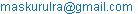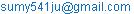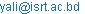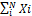Science Journal of Applied Mathematics and Statistics
Volume 3, Issue 5, October 2015, Pages: 230-233

Selection of the Samples with Probability Proportional to Size

Maskurul Alam, Sharmin Akter Sumy, Yasin Ali Parh

Department of statistics, Islamic University, Kushtia, Bangladesh(M. Alam)(S. Sumy)(Y. Parh)

Maskurul Alam, Sharmin Akter Sumy, Yasin Ali parh. Selection of the Samples with Probability Proportional to Size.Science Journal of Applied Mathematics and Statistics.Vol.3, No. 5, 2015, pp. 230-233.
doi:
10.11648/j.sjams.20150305.13

Abstract: It is manifested to all that sample size varies from unit to unit. It goes without saying that large units contain more apropos information than the smaller units. So if the unit size is larger then there is a greater possibility to choose sample from the large unit than smaller one. It actually means the probability of selecting a unit is positively proportional to its sizes. The selection of unit is done corresponding to choose a number at random from the totality of numbers associated. My main aim is to prefer a method of selecting units on the basis of its size.

Keywords: The Probability Proportional to Size (PPS), Cumulative Method, Lahiri’S Method

1. Introduction

In simple random sampling the chance of each selecting units are equal. But often units may vary in sizes. If simple random sampling is being used in this case the expected result or desired information may not get. Under this circumstance, such subordinate information can be utilized in selecting the sample so as to more precise estimators of the population parameters. The probabilities of selecting samples to different units depend on their sizes. The probability of selection may be assigned to the proportional of the sizes are known to us probability proportion to sampling size (PPS).

Let consider y is the variable under study. We are considering Y is the shopping malls in a town and X is an auxiliary variables or number of workers who works in these factories. The most commonly used varying probability scheme. The shopping malls are selected with proportional to their number of workers. This term is known to us Probability proportion to sampling (PPS).

2. PPS Sampling Procedure with Replacement

We are discussing here the two methods to draw sample PPS with replacement.

2.1. The Cumulative Total Method

Let the size of the unit be Xi (i=1, 2, 3 …N). The total being X=. We associate the numbers 1 to X1 with the first unit. Then we associate the numbers (X1+1) to (X1+X2) with second unit, the numbers (X1+X2+1) to (X1+X2+X3) with third unit and so on. A number K is being chosen at random from 1 to X. Now we observe the unit with which this random number is concomitant is selected. Clearly the ith units are being selected with probability proportional to Xi. It is clearly can be stated that if a sample of size n is required, the procedure is repeated n times with replacement of the units selected.

2.2. Cumulative Total Method on the Basis of Practical Example

Table 1. The data set of the workers in the shopping mall and their output.

 Shopping mall no Number of workers sizes Sell commodities Cumulative totals 1 2 30 C1=2 2 5 60 C2=2+5=7 3 11 12 C3=7+11=18 4 13 6 C4=18+13=31 5 7 8 C5=31+7=38 6 3 13 C6=38+3=41 7 9 4 C7=41+9=50 8 16 17 C8=50+16=66 9 6 13 C9=66+6=72 10 4 8 C10=72+4=76

We will describe the cumulative total method on the basis of example. We consider a district Kushtia that contains 10 shopping malls and where following number of workers work 2, 5, 11, 13,7,3,9,16,6 and 4. We select a sample of four shopping malls with replacement method for knowing the life pattern of workers.

The first step of selecting factories is to form cumulative totals. So we will determine the cumulative totals in order to compare with selected random numbers.

2.3. Selection of Sample Using Cumulative Total Method

It is necessary to bear in mind that that To select 4 shopping malls, we have to choose such random numbers from 1 to 76 that don’t exceed 76.

First draw:

Suppose we select a random number k=23. It can be seen from the cumulative total that this selected random number associated with C4. So unit Y is selected and Y4=6.

Second Draw:

Now further we select a random number k=38. It can be easily seen from above table that it associated with C5. So clearly the shopping mall Y5=8 is being selected corresponding to the random number 38.

Third and fourth Draw:

Similarly, we select two more random numbers from random table. Suppose these numbers are 15 and 45. Then the selected shopping malls are c3=12 and c6=13 corresponding to its random numbers. Now we could make analysis or our desire work on these selected 4 shopping malls.

So at length we may deduce from here that the probability of selecting unit in this procedure is proportional to its size.

Drawback:

The major draw backs of cumulative total is finding successive cumulative total must be found. This is time consuming process and wearisome.

This problem could be solved by the help of Lahiri’s method.

3. Lahiri’s Method

Let M=max Xi i.e. maximum of the sizes of N units in the population or some convenient number greater than M. We can write the following steps in a nutshell in order to select our desire samples:

Select a pair of random number (i, j) such that 1≤i≤N and 1≤j≤M

If j ≤ Xi then ith unit is selected otherwise rejected and another pair of random number is chosen.

To get a sample of size n, this procedure is repeated till n units are selected.

3.1. Lahiri’s Method on the Basis of Practical Example

We’ll describe the Lahiri’s method on the basis of practical instance. This is an alternative procedure in which cumulation is avoided wholly. We consider the previous example of shopping malls of Kushtia district. We select a sample of four shopping malls by Lahiri’s method of PPS.

Table 2. The data set of the workers in the shopping mall and their output without cumulative total.

 Shopping mall no Number of workers Sell commodities Cumulative total are avoided. 1 2 30 2 5 60 3 11 12 4 13 6 5 7 8 6 3 13 7 9 4 8 16 17 9 6 13 10 4 8

3.2. Selection of Sample Using Lahiri’s Method

The given number of shopping malls N=10, First of all we select the random number between 1-N. It means we have to choose such random numbers those are less than 10. Suppose 3 is selected. It is noting down that unit with corresponding serial number provisionally selected. We select another random number between 1 to M where M= Max Xi=16. Suppose our second random number 7 is selected. Now, if the second random number that we have selected smaller than the size of unit provisionally selected. Then the unit is selected into the sample. If not then entire procedure will be repeated until is finally selected. We are considering these selected random numbers into table.

Table 3. The table of selected and rejected shopping mall.

 1st random numbers 1≤i≤10 2nd random numbers 1≤j≤16 Observation Selection Shopping malls 3 7 j=7 3rd number shopping malls selected 6 13 J=13>X6=3 rejected 4 5 j=5 4rth number shopping mall selected. 2 3 J=3 2nd number selected 9 7 J=7>X9=6 Rejected 7 8 J=8 7th number shopping mall selected

The pairs (3,7),(4,5),(2,3),(7,8) are selected. Hence the samples will consist of the shopping malls with serial number 3, 4, 2 and 7

The sum and substance of this method is that we will repeat the procedure until our desire samples are selected.

The basic difference between simple random sampling and varying probability scheme:

In simple random sampling the probability of selecting unit at any drawn is the same. But in varying probability scheme the probability of selecting any unit differ from unit to unit. It appears in PPS sampling that such procedure would give biased estimators as the larger units are over-represented and the smaller units are under-represented in the sample. This will happen in case of sample mean as an estimator of population mean.

4. The Cumulative Total Method for without Replacement

For selecting a sample of size n without replacement, the first unit is selected by the above cumulative total method and then it is deleted from the population and for the reminder population new cumulative totals are calculated and again the same procedure is used to select a second unit. The procedure is continued until a sample of n units is obtained.

The procedure is illustrated in table. We consider the first example for explaining the cumulative total method for without replacement. We will describe the cumulative total method on the basis of example. We consider a district Kushtia that contains 10 shopping malls and where following number of workers work 2, 5, 11, 13,7,3,9,16,6 and 4. We select a sample of 2 factories without replacement method for knowing their life pattern.

The first step of selecting factories is to form cumulative totals. So we will determine the cumulative totals in order to compare with selected random numbers.

Table 4. The data set of 10 numbers of workers in the shopping mall and their output.

 Shopping mall no Number of workers sizes Sell commodities Cumulative totals 1 2 30 C1=2 2 5 60 C2=2+5=7 3 11 12 C3=7+11=18 4 13 6 C4=18+13=31 5 7 8 C5=31+7=38 6 3 13 C6=38+3=41 7 9 4 C7=41+9=50 8 16 17 C8=50+16=66 9 6 13 C9=66+6=72 10 4 8 C10=72+4=76

Suppose we wish to draw a pps sample of 3 factories without replacement for the selection of the first unit. We choose such random numbers that don’t exceed 76. We select a random number k=37. We could see from the table it lies in 6th no unit. So it will be selected. Now we remove this unit and rearrange the shopping mall and calculate the cumulative total.

Table 5. The rearrangement of shopping mall and their output.

 Shopping mall no Number of workers sizes Sell commodities Cumulative totals 1 2 30 2 2 5 60 7 3 11 12 18 4 13 6 31 5 7 8 38 7 9 4 47 8 16 17 62 9 6 13 68 10 4 8 72

Now for the second draw we select another unit that doesn’t exceed 72. So we select a random number, suppose it is 65 and lies in 9th unit. So 9th unit will be selected then again we remove this selected unit and rearrange the unit and calculate the cumulative total.

Tbale 6. The rearrangement of shopping mall and their output for the new unit.

 Shopping mall no Number of workers sizes Sell commodities Cumulative totals 1 2 30 2 2 5 60 7 3 11 12 18 4 13 6 31 5 7 8 38 7 9 4 47 8 16 17 62 10 4 8 66

Thus the PPS sample of 2 shopping malls without replacement consists of shopping mall with serial numbers 6 and 9.

The drawback:

The main disadvantage of cumulative total method is that it involves writing down of the cumulative total Ti which is tedious and time consuming.

The procedure of selection of a pps sample by using Lahiri’s method:

For selection of PPS of n units without replacement the units selected in the earlier draws are removed from the population. From the remaining units another PPS sample of size one is taken as before and the selected unit removed from the population. This procedure is repeated until n selections are made. In this case Lahiris’s method is being used.

5. The Practical Example of Choosing Samples by Using Lahiri’s Method

We consider previous example of shopping mall. Suppose we wish to select 2 shopping malls with probability proportional to the number of workers in the shopping malls without replacement.

We will select a pair of random numbers (i, j), (i≤10, j≤16). Where i are the number of units (shopping malls) and j are the sizes(number of works in different shopping malls). Now using the random number table we get the pair (3, 10). Since the number of workers Xi for shopping mall 3 is greater than the second number 10 of the selected random pair. So the 3rd shopping mall is selected in the sample. For selecting the second unit by probability proportional to the number of workers in the shopping malls, we prepare the following arrangement once again after deleting the 3rd shopping mall (unit).

As in pair (3, 10), a pair of random numbers (i, j), (i≤9, j≤16) has to be selected, using the random number table , referring to the table of random numbers, the pair selected is (4,6). As the size of the 4 unit in the above arrangement is greater than the second number of pair random number, so the shopping mall 4 will be selected into the sample.

Table 7. The rearrangement of shopping mall and their output for Lahiri’s method.

 Shopping mall no Number of workers Sell commodities Cumulative total are avoided. 1 2 30 2 5 60 3 13 6 4 7 8 5 3 13 6 9 4 7 16 17 8 6 13 9 4 8

6. Conclusion

It is known to us that Hansen and Hurwitz first introduced the use of probability proportional to size (PPS) sampling; it goes without saying that a number of procedures for selecting samples without replacement have been developed by the help of statisticians. Survey statisticians have found probability proportional to size (PPS) sampling scheme more useful for selecting units from the population as well as estimating parameters of interest especially when it is clear that the survey is large in size and involves multiple characteristics. So finally we could say that the selection of samples are being done on the basis of its unit sizes.

References

1. Amahia GN, Chaubey YP, Rao TJ (1989). Efficiency of a new PPS sampling for multiple characteristics, J. Stat.Plan. Inference, 21, 75-84.
2. Basu D (1971). An essay on the logical foundation of survey sampling I" Foundation of statistical inference.. Holt, Rinehard and Winston. Edited by Godambe and Sprott
3. Brewer, K. R. W. and Hanif, M. (1983). Sampling with unequal probabilities, New York: Springer-Verlag.
4. Chaudhuri A (2010). Essentials of Survey Sampling, PHI Learning Private Limited, New Delhi.
5. Ekaette, IE, (2008). A class of alternative estimators for Multicharacteristics in PPS sampling Scheme, Unpublished Ph.D thesis, Univeristy of Ibadan, Nigeria.
6. Godambe VP (1955). A unified theory of sampling from finite population, J. Roy. Stat. Soc. B., 17, 269- 278.
7. Grewal IS, Bansal ML, Singh (1997). An alternative estimator for multiple characteristics using randomized response technique in PPS sampling. Aligarh J. Stat., 19:51-65.
8.  8 Hansen MH, Hurwitz WN (1943). On the theory of sampling from a finite population, Ann. Math. Stat., 14, 333-362.
9. Hansen, Morris H., William N. Hurwitz and William G. Madow., (l953b).  Sample Survey Methods and Theory, Volume 2- Theory, New York. John Wiley and Sons.
10. Kendall, M. G., and Smith, B. B., (1938). Randomness and random sampling numbers, Jour. Roy. Stat. Soc., 101, 147-166.
11. Pathak PK (1966). An estimator in PPS sampling for multiple characteristics. Sankhya, A. 28(1):35-40. Rand Corporation (1955). A Million Random Digits, Free Press, Glencoe, III.
12. Rao JNK (1966). Alternative estimators in the PPS sampling for multiple characteristics,  Sankhya, 28(A), 47-60.
13.  Singh S, Grewal IS, Joarder A (2004). General class of estimators in multi-character surveys. Stat. Papers, 45:571-582.

 Contents 1. 2. 2.1. 2.2. 2.3. 3. 3.1. 3.2. 4. 5. 6.
Article ToolsAbstractPDF(188K)